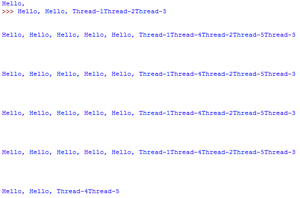Open in App
Not now

# Synchronization by using Semaphore in Python

• Last Updated : 11 Oct, 2020

In Lock and RLock, at a time only one Thread is allowed to execute but sometimes our requirement is to execute a particular number of Threads at a time.

Suppose we have to allow at a time 10 members to access the Database and only 4 members are allowed to access Network Connection. To handle such types of requirements we can not use Lock and RLock concept and here we should go for Semaphore. Semaphore can be used to limit the access to the shared resources with limited capacity. It is an advanced part of synchronization.

Create an object of Semaphore:

```object_name = Semaphore(count)
```

Here ‘count’ is the number of Threads allowed to access simultaneously. The default value of count is 1.

When a Thread executes acquire() method then the value of “count” variable will be decremented by 1 and whenever a Thread executes release() method then the value of “count” variable will be incremented by 1. i.e whenever acquire() method will be called the value of count variable will be decremented and whenever release() method will be called the value of “count” variable will be incremented.

Way to create an object of Semaphore :

Case 1 :

`object_name.Semaphore()`

In this case, by default value of the count variable is 1 due to which only one thread is allowed to access. It is exactly the same as the Lock concept.

Case 2

`object_name.Semaphore(n) `

In this case, a Semaphore object can be accessed by n Threads at a time. The remaining Threads have to wait until releasing the semaphore.

Executable code of Semaphore :

## Python3

 `# importing the modules ` `from` `threading ``import` `*`          `import` `time         ` ` `  `# creating thread instance where count = 3 ` `obj ``=` `Semaphore(``3``)         ` ` `  `# creating instance ` `def` `display(name):     ` `   `  `    ``# calling acquire method ` `    ``obj.acquire()                 ` `    ``for` `i ``in` `range``(``5``): ` `        ``print``(``'Hello, '``, end ``=` `'') ` `        ``time.sleep(``1``) ` `        ``print``(name) ` `         `  `        ``# calling release method ` `        ``obj.release()     ` `         `  `# creating multiple thread  ` `t1 ``=` `Thread(target ``=` `display , args ``=` `(``'Thread-1'``,)) ` `t2 ``=` `Thread(target ``=` `display , args ``=` `(``'Thread-2'``,)) ` `t3 ``=` `Thread(target ``=` `display , args ``=` `(``'Thread-3'``,)) ` `t4 ``=` `Thread(target ``=` `display , args ``=` `(``'Thread-4'``,)) ` `t5 ``=` `Thread(target ``=` `display , args ``=` `(``'Thread-5'``,)) ` ` `  `# calling the threads  ` `t1.start() ` `t2.start() ` `t3.start() ` `t4.start() ` `t5.start()`

Output:Here in the above example first we created an instance of Semaphore Class where the value of “count” is 3 it means that Semaphore Object can be accessed by 3 Threads at a time. Now we have created display() method which will print the Thread name 5 times. Now we created 5 threads and now whenever we call start() method at that time 3 Threads are allowed to access Semaphore objects and hence 3 Threads are allowed to execute display() method at a time but in this example whenever we will execute we will get irregular output because 3 Threads are executing display() method at a time.

My Personal Notes arrow_drop_up
Related Articles